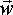# Maths - Skew Symmetric Matrix

A matrix is skew symmetric if its elements meet the following rule:

aij = - aji

The leading diagonal terms must be zero since in this case a= -a which is only true when a=0.

We can express this as:

[A]t = -[A]

## Representing Vector cross Multiplication

We want to find a 3x3 matrix which is equivalent to vector cross multiplication (as described here). Note: cross multiplication only applies to 3 dimensional vectors and represents a vector which is perpendicular to both the vectors being multiplied.

In other words, if,

C = A × B

where:

• A,B and C are 3 dimensional vectors
• × is the cross product

Then we want to find:

C = [~A] B

where:

[~A] = 3x3 Skew Symmetric Matrix which we want to find

The components of the cross product are (as described here):

Cx = Ay * Bz - By * Az
Cy = Az * Bx - Bz * Ax
Cz = Ax * By - Bx * Ay

So [~A] is equivalent to A x

[~A] =
 0 -Az Ay Az 0 -Ax -Ay Ax 0

## Properties of Skew Symmetric Matrix

### Jacobis theorem

There is no inverse of skew symmetric matrix in the form used to represent cross multiplication (or any odd dimension skew symmetric matrix), if there were then we would be able to get an inverse for the vector cross product but this is not possible.

To demonstrate this we can try to calculate its inverse as follows:

We can use the expression for inverse given here and apply it to skew symmetric matrix to get:

[~A] =1/det[m] *
 Ax*Ax Ay*Ax Az*Ax Ax*Ay Ay*Ay Az*Ay Ax*Az Ay*Az Az*Az

where det = Ay*Az*Ax -Az*Ax*Ay = 0

So all terms tend towards 1/0 = infinity

## 'tilde' matrix

If we have a vector cross multiplication, for instance:=xWe can replace this expession by an equivilant matrix transform:= [~r]Where [~r] is a square matrix derived as follows, if we expand out the terms for vector cross multiplation we get:

vx = ry * wz - wy * rz
vy = rz * wx - wz * rx
vz = rx * wy - wx * ry

we can rearange these terms to be in matrix form as follows:

vx = 0 * wx - rz* wy + ry * wz
vy = rz * wx + 0 * wy - rx * wz
vz =-ry * wx + rx * wy + 0 * wz

which gives:=
 0 - rz ry rz 0 - rx -ry rx 0so

[~r] =
 0 - rz ry rz 0 - rx -ry rx 0

If instead ofwe want to turninto the matrix, then we can use the following identiy for vectors (reversing the order of operands changes the sign) :x= - (x)

Therefore if=xthen= -[~w], where:

-[~w] =
 0 wz -wy -wz 0 wx wy -wx 0

 metadata block see also: Correspondence about this page Book Shop - Further reading. Where I can, I have put links to Amazon for books that are relevant to the subject, click on the appropriate country flag to get more details of the book or to buy it from them.Mathematics for 3D game Programming - Includes introduction to Vectors, Matrices, Transforms and Trigonometry. (But no euler angles or quaternions). Also includes ray tracing and some linear & rotational physics also collision detection (but not collision response). Other Math Books Specific to this page here:

This site may have errors. Don't use for critical systems.